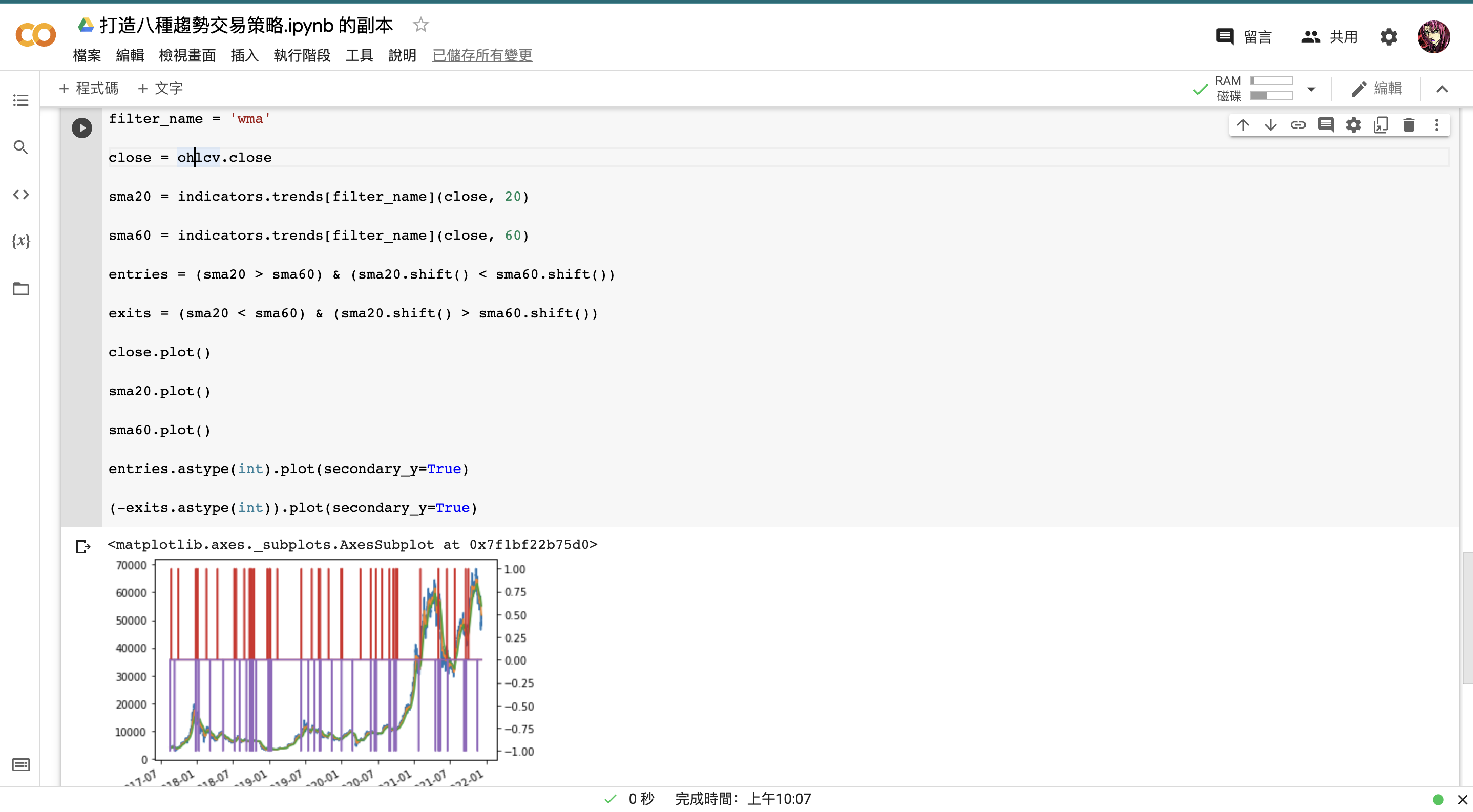# 第二章：1 打造八種趨勢交易策略

（請參考第一章第一節來啟動該筆記本）

### b. 單 filters 撰寫

import pandas as pd

s = pd.Series( * 200 +  * 300 +  * 200)

s.plot()


from finlab_crypto import indicators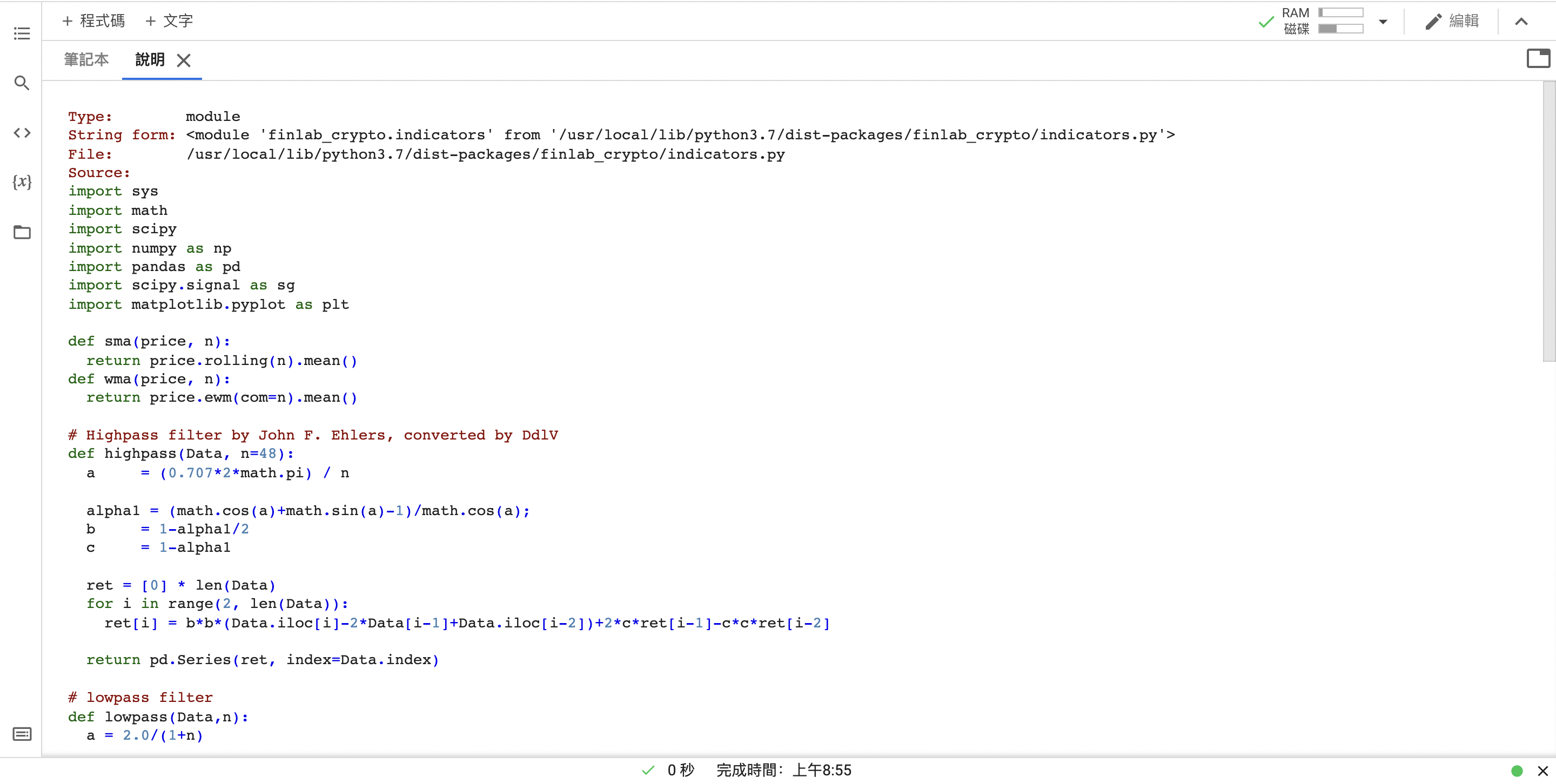trend = indicators.trends['sma'](s, 50)

s.plot()

trend.plot()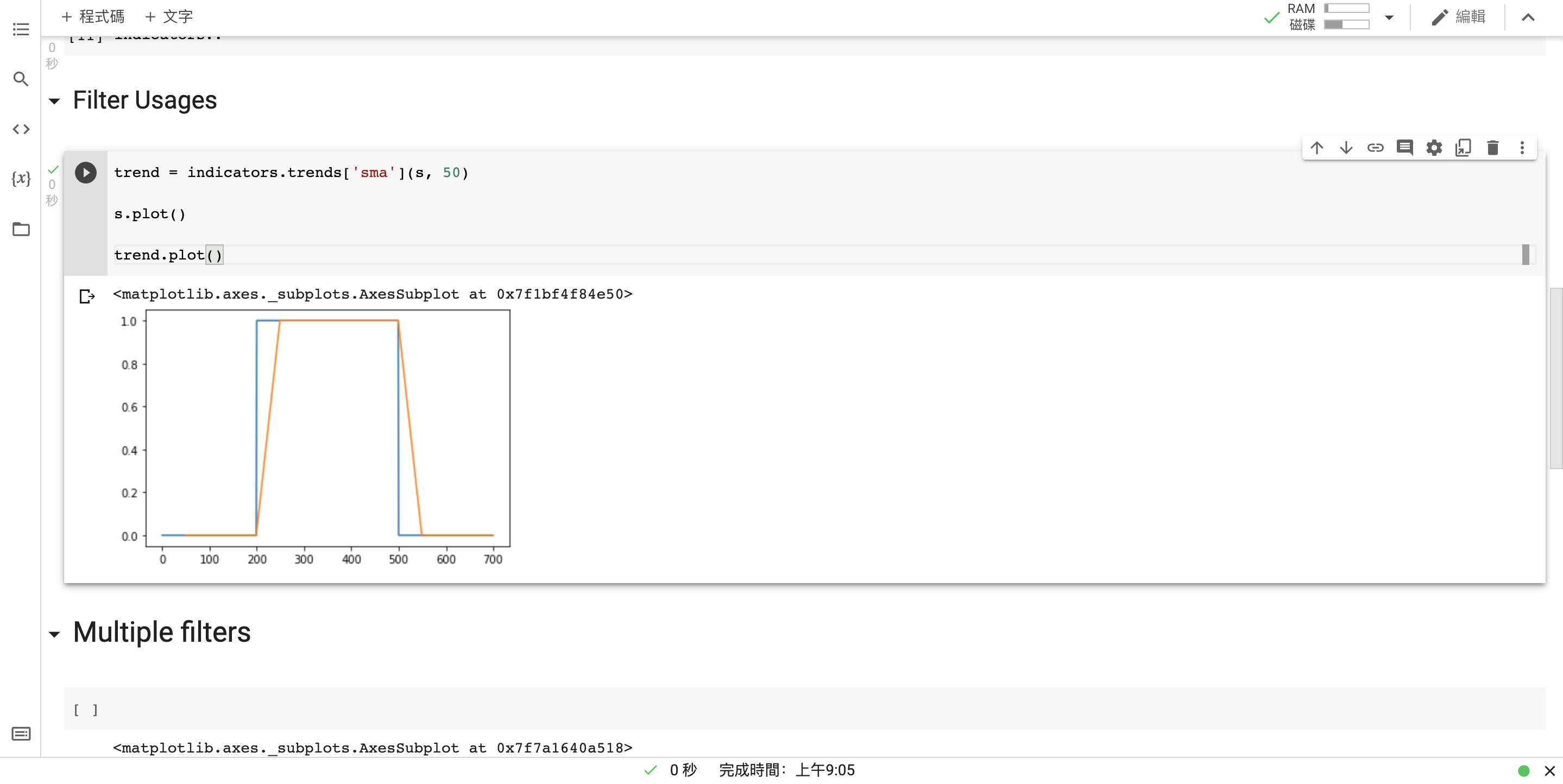### c. 多 filters 撰寫

for name, func in indicators.trends.items():

print(name, func)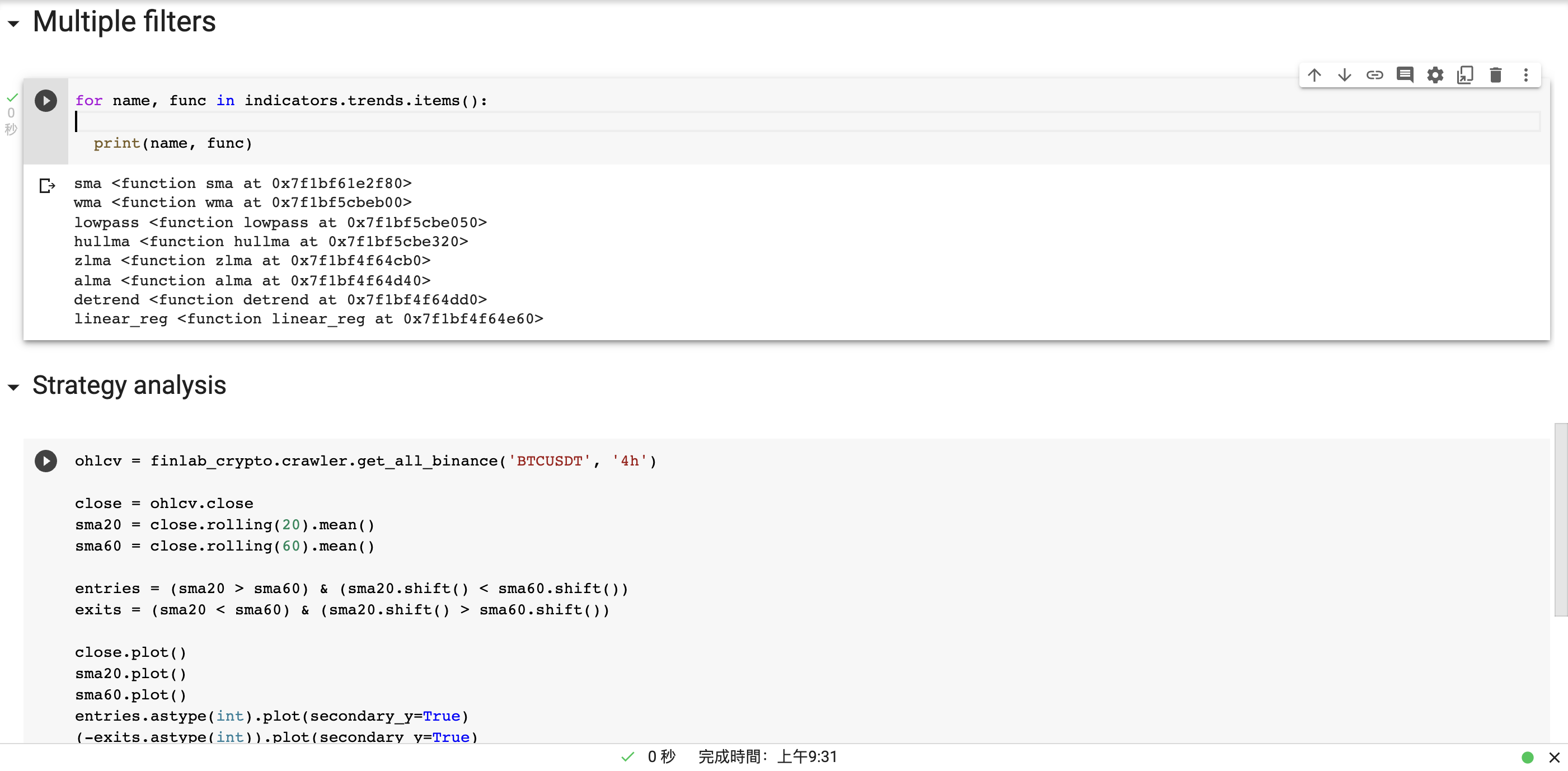s.plot()

for name, func in indicators.trends.items():

trend = func(s, 50)

trend.plot()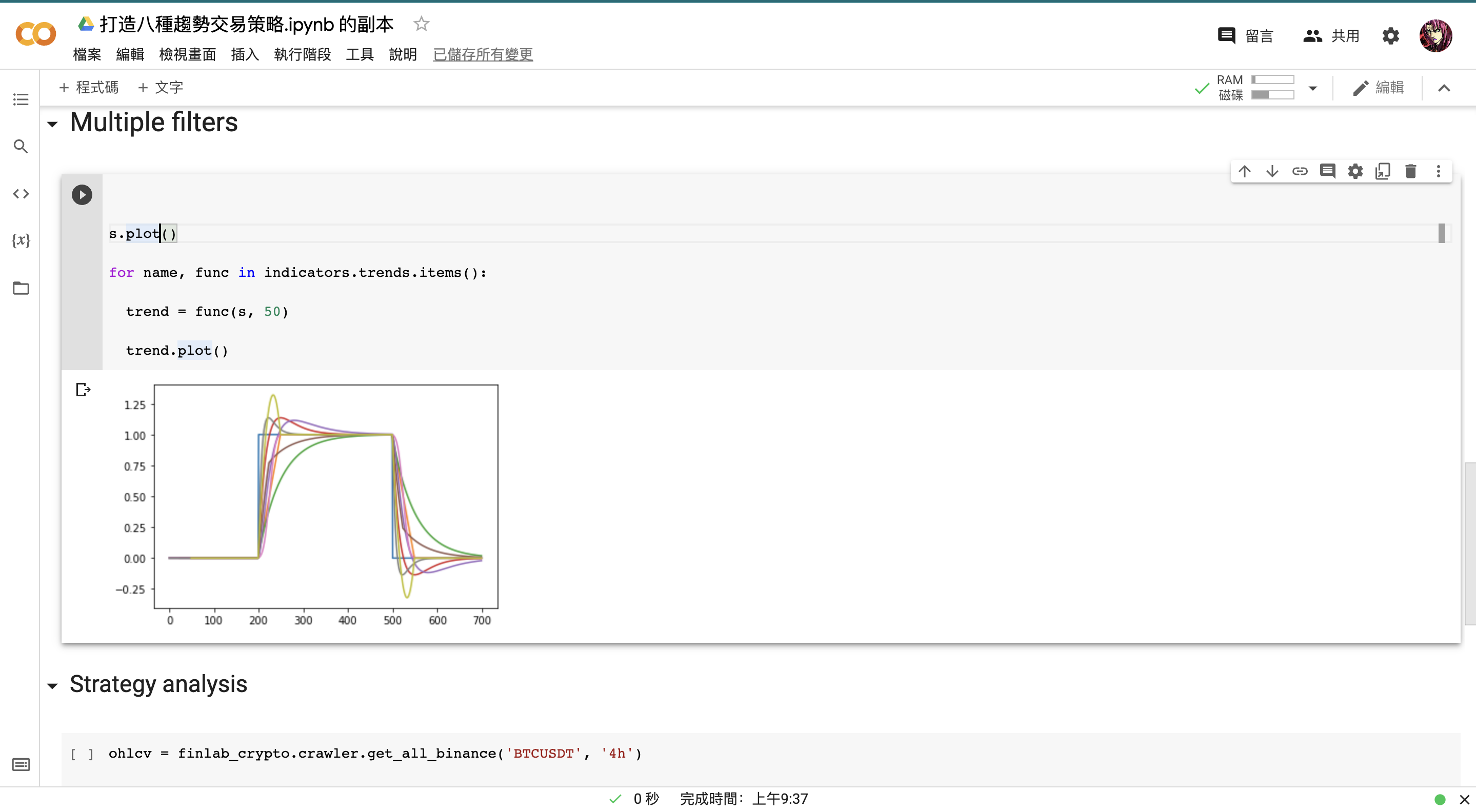filter_results = pd.DataFrame()

filter_results['original_price'] = s

for name, func in indicators.trends.items():

trend = func(s, 50)

filter_results[name] = trend

filter_results.plot()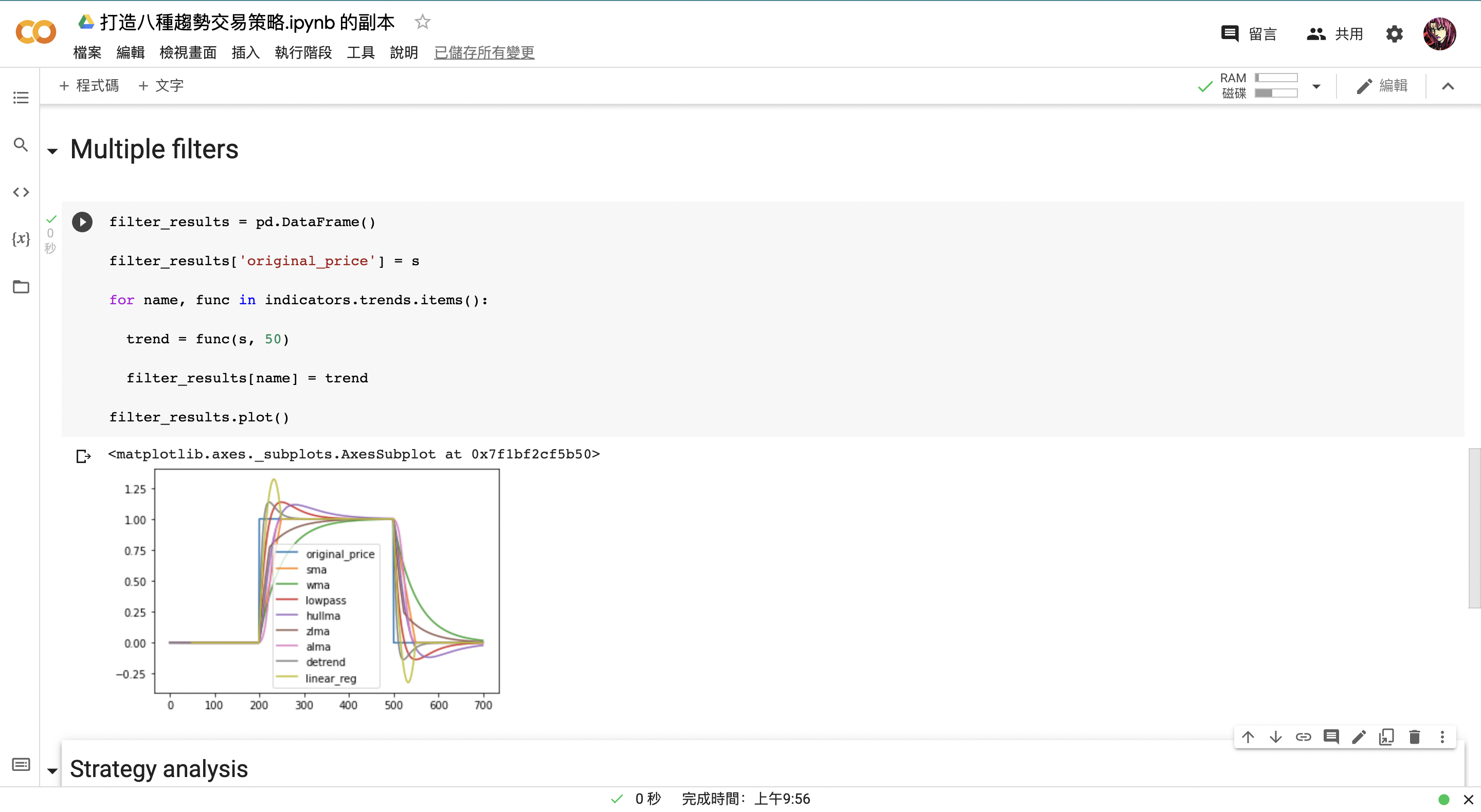### d. 撰寫交易策略

ohlcv = finlab_crypto.crawler.get_all_binance('BTCUSDT', '4h')


close = ohlcv.close

sma20 = close.rolling(20).mean()

sma60 = close.rolling(60).mean()

entries = (sma20 > sma60) & (sma20.shift() < sma60.shift())

exits = (sma20 < sma60) & (sma20.shift() > sma60.shift())

close.plot()

sma20.plot()

sma60.plot()

entries.astype(int).plot(secondary_y=True)

(-exits.astype(int)).plot(secondary_y=True)


filter_name = 'wma'

close = ohlcv.close

sma20 = indicators.trends[filter_name](close, 20)

sma60 = indicators.trends[filter_name](close, 60)

entries = (sma20 > sma60) & (sma20.shift() < sma60.shift())

exits = (sma20 < sma60) & (sma20.shift() > sma60.shift())

close.plot()

sma20.plot()

sma60.plot()

entries.astype(int).plot(secondary_y=True)

(-exits.astype(int)).plot(secondary_y=True)